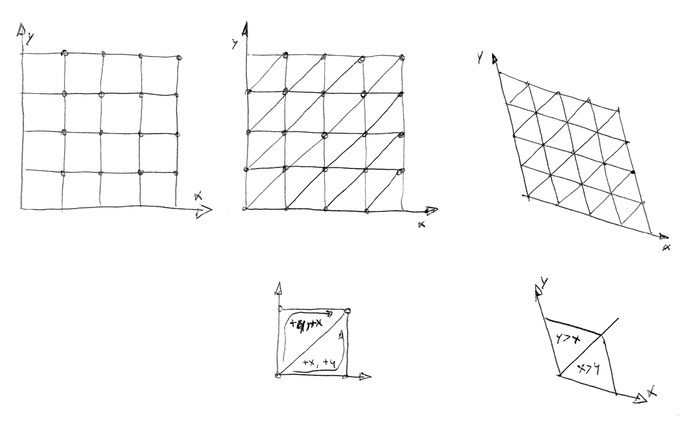## 随机

—— https://zh.wikipedia.org/wiki/随机性

• 随机数：即随机产生的数字，计算机中常用的随机算法都是产生一个[0, 1)之间的数；随机数最重要的特性是它在产生时后面的那个数与前面的那个数毫无关系
• 伪随机（确定性随机）：即通过一个固定的计算函数来获取一个『随机』数，只要初值（随机种子）相同，得到的随机数就是相同的，得到随机数就是伪随机数，但是它们具有类似于随机数的统计特征

### 伪随机函数

• 足够混乱（相邻的种子数得到的『随机数』应该有较大差别
• 周期应该比较长
• 不太容易预测出『随机值』（虽然确实有具体的函数描述）

#### 三角函数

1. 使周期变小，小到无法分辨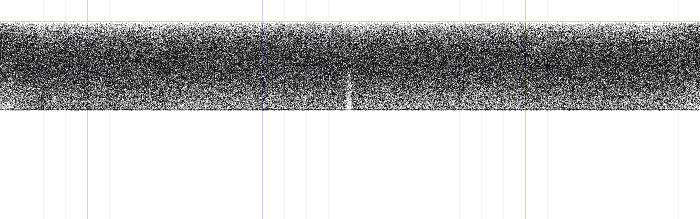上面这个图像是函数$y = |sin(x * 545673.42)|$生成的随机数图像，可以看到取得周期数极小，得到的随机图像也是『杂乱无章』，但是如果周期数与当前系统的最小精度之间存在倍数关系时，就会出现很有规律的随机值分布，如$y = |sin(x * 4153745.42)|$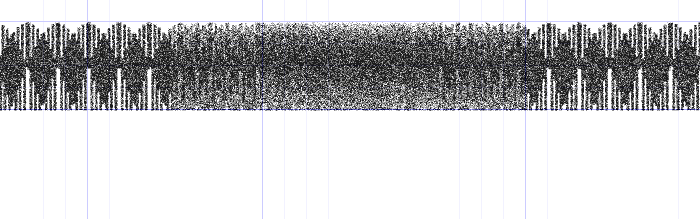2. 使长度变大，截取小数部分（相当于放大小数部分）；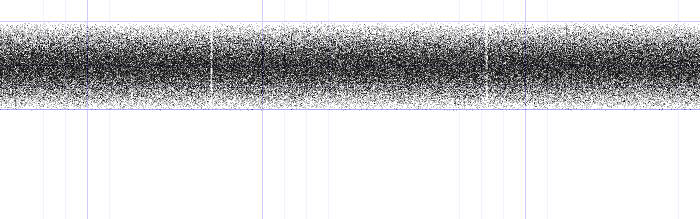上述图像是函数$y = fract(sin(x) * 85595.42)$得到的随机数分布图像；可以很明显的看到在函数极值处有比较明显的分割；且这种方法也同样存在第一种方法的问题，即放大倍数过大时，以至于得到的随机数分布出现比较规律的变化，如$y = fract(sin(x) * 23485595.42)$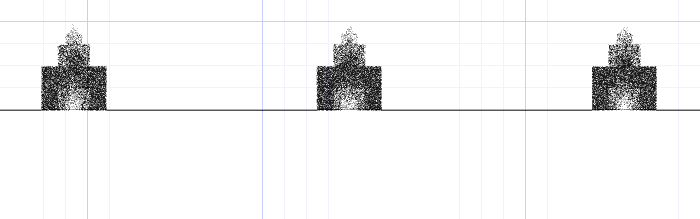3. 两种方法结合可以避免一些问题：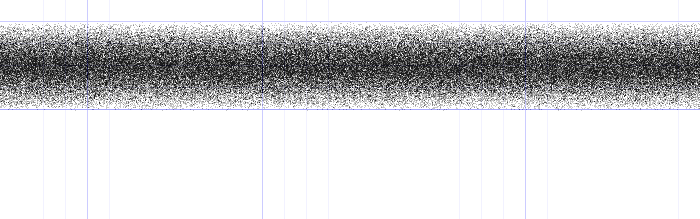函数为：$y = fract(sin(x / 84.462) * 59595.42)$；通过同时放大小数部分，提高函数周期，可以使得到的随机数分布更均匀，更『随机』；

#### 取小数部分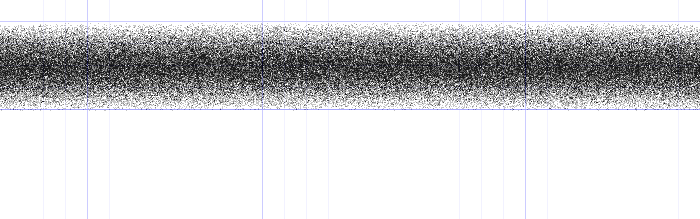## 噪声

### 一维线性插值

$c = a + k * (b - a)$

### 一维多项式插值

$\begin{cases} u &= \displaystyle\sum_{i=1}^n{p_i * k^i},\ (p_i\text{是对应项的系数}) \\ c &= a + u * (b - a) \end{cases}$

### 三次多项式插值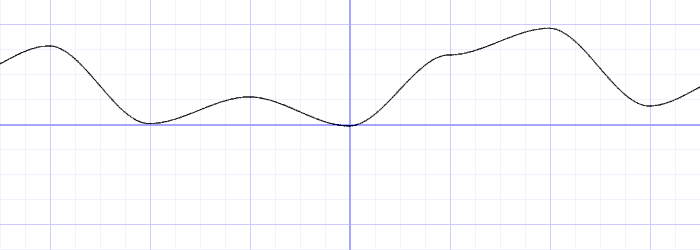$u(k) = 3*k^2 - 2*k^3$

### Perlin 噪声

Perlin噪声满足以下特征

• 对旋转具有统计不变性
• 能量在频谱上集中于一个窄带，即：图像是连续的，高频分量受限；
• 对变换具有统计不变性。

• 三次多项式：

$u(k) = 3*k^2 - 2*k^3$

• 五次多项式：

$u(k) = 6*k^5 - 15*k^4 + 10 * k^3$

### 二维插值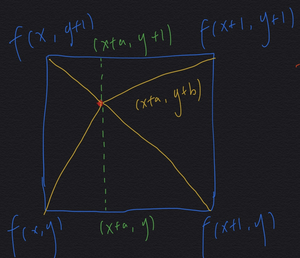$f'(x + a, y + b) = \begin{bmatrix} 1 - u(a) & u(a) \end{bmatrix} \begin{bmatrix} f(x, y) & f(x, y + 1) \\ f(x + 1, y) & f(x + 1, y + 1) \end{bmatrix} \begin{bmatrix} 1 - u(b) \\ u(b) \end{bmatrix}$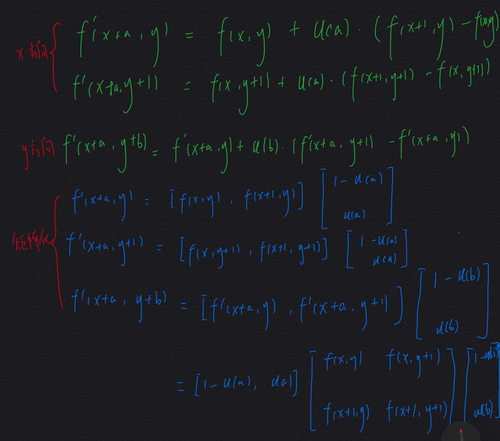### Simplex 噪声

Simplex噪声是Ken Perlin（没错，就是Perlin噪声的提出者）提出的更自然，性能更好的噪声算法。

• 在二维插值的时候，不使用正方形的四个顶点进行插值，而是使用等边三角形的三个顶点进行插值；更高维的插值也是如此，也就是说N维的插值只需要N+1个点！比传统的指数型增长相比改善显著！

• 使用五次多项式进行插值，使边界处的衔接更平滑